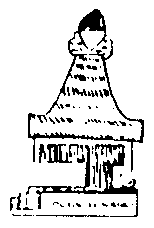# Tendency of the dayThe Tendency of the Day is calculated by adding the Fundamental Tendency to the

kabahstic sum of the date we have chosen

By means of this system one knows how things will go so as to know how to act on that

Day.

1.- EXAMPLE: Victor Manuel Chavez : Day, 30 April 1969.

Fundamental Tendency: 5.

Day 30 = 3 + 0 = 3

Month 4

Year 1969 = 1 + 9 + 6 + 9 = 25 => 2 + 5 = 7

Day 3

Month 4

Year 7

14 => 14 = 1 + 4 = 5

5 + 5 (Fundamental Tendency) = 10.

10 = 1 + 0 = 1 Tendency of the Day 30-4-1969

The 10 is the Wheel of Fortune change The 1 is initiative, that which begins, that which

Commences, originality, effort.

2.- EXAMPLE: Mr Guillermo Hickie Tendency for 1-5-1969

Fundamental Tendency 8

Day 1

Month 5

Year 1969 = 1 + 9 + 6 + 9 = 25 => 2 + 5 = 7

Day 1

Month 5

Year 7

13 Death = 1 + 3 = 4 => 4 + 8 (Fundamental Tendency) = 12

12 = 1 + 2 = 3 => Tendency of the Day 1-5-1969.

The 3 is the artistic, the Three Primary Forces, material and spiritual production.

Production, increase, the beautiful, the creative, harmony, art, beauty.

3.- EXAMPLE: Mr Gil: Tendency of 27 July, 1969.

Fundamental Tendency: 3

Day 27 = 2 + 7=9

Month 7

Year 1969 1 + 9 + 6 + 9 = 25 => 2 + 5 = 7

Day 9

Month 7

Year 7

23 = 2 + 3 = 5 => 5 + 3: Fundamental Tendency = 8

The 8 is tests, suffering. Patience, cooperation must be increased, to know how to wait.

To reflect, seriousness of thought, to distinguish between the most and the least useful,

between that which is, and that which is not. To not do things madly to scrutinise the pros

and cons with patience.

Each one of the numbers of the Kabalah must be studied in depth.